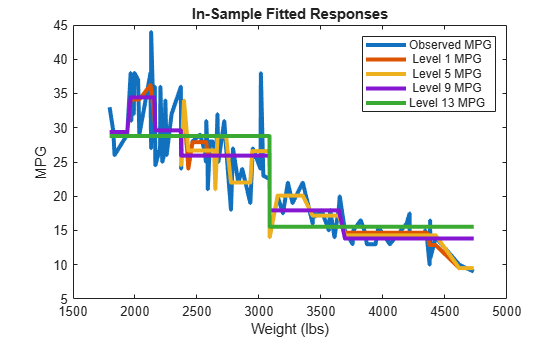# resubPredict

Class: RegressionTree

Predict resubstitution response of tree

## Syntax

```Yfit = resubPredict(tree) [Yfit,node] = resubPredict(tree) [Yfit,node] = resubPredict(tree,Name,Value) ```

## Description

`Yfit = resubPredict(tree)` returns the responses `tree` predicts for the data `tree.X`. `Yfit` is the predictions of `tree` on the data that `fitrtree` used to create `tree`.

```[Yfit,node] = resubPredict(tree)``` returns the node numbers of `tree` for the resubstituted data.

```[Yfit,node] = resubPredict(tree,Name,Value)``` predicts with additional options specified by one or more `Name,Value` pair arguments.

## Input Arguments

expand all

 `tree` A regression tree constructed using `fitrtree`.

### Name-Value Arguments

Specify optional pairs of arguments as `Name1=Value1,...,NameN=ValueN`, where `Name` is the argument name and `Value` is the corresponding value. Name-value arguments must appear after other arguments, but the order of the pairs does not matter.

Before R2021a, use commas to separate each name and value, and enclose `Name` in quotes.

Pruning level, specified as the comma-separated pair consisting of `'Subtrees'` and a vector of nonnegative integers in ascending order or `'all'`.

If you specify a vector, then all elements must be at least `0` and at most `max(tree.PruneList)`. `0` indicates the full, unpruned tree and `max(tree.PruneList)` indicates the completely pruned tree (i.e., just the root node).

If you specify `'all'`, then `resubPredict` operates on all subtrees (i.e., the entire pruning sequence). This specification is equivalent to using `0:max(tree.PruneList)`.

`resubPredict` prunes `tree` to each level indicated in `Subtrees`, and then estimates the corresponding output arguments. The size of `Subtrees` determines the size of some output arguments.

To invoke `Subtrees`, the properties `PruneList` and `PruneAlpha` of `tree` must be nonempty. In other words, grow `tree` by setting `'Prune','on'`, or by pruning `tree` using `prune`.

Example: `'Subtrees','all'`

Data Types: `single` | `double` | `char` | `string`

## Output Arguments

 `Yfit` The response `tree` predicts for the training data. If the `Subtrees` name-value argument is a scalar or is missing, `label` is the same data type as the training response data `tree.Y`. If `Subtrees` contains `m`>`1` entries, `label` has `m` columns, each of which represents the predictions of the corresponding subtree. `node` The `tree` node numbers where `tree` sends each data row. If the `Subtrees` name-value argument is a scalar or is missing, `node` is a numeric column vector with `n` rows, the same number of rows as `tree.X`. If `Subtrees` contains `m`>`1` entries, `node` is a `n`-by-`m` matrix. Each column represents the node predictions of the corresponding subtree.

## Examples

expand all

Load the `carsmall` data set. Consider `Displacement`, `Horsepower`, and `Weight` as predictors of the response `MPG`.

```load carsmall X = [Displacement Horsepower Weight];```

Grow a regression tree using all observations.

`Mdl = fitrtree(X,MPG);`

Compute the resubstitution MSE.

```Yfit = resubPredict(Mdl); mean((Yfit - Mdl.Y).^2)```
```ans = 4.8952 ```

You can get the same result using `resubLoss`.

`resubLoss(Mdl)`
```ans = 4.8952 ```

Load the `carsmall` data set. Consider `Weight` as a predictor of the response `MPG`.

```load carsmall idxNaN = isnan(MPG + Weight); X = Weight(~idxNaN); Y = MPG(~idxNaN); n = numel(X);```

Grow a regression tree using all observations.

`Mdl = fitrtree(X,Y);`

Compute resubstitution fitted values for the subtrees at several pruning levels.

```m = max(Mdl.PruneList); pruneLevels = 1:4:m; % Pruning levels to consider z = numel(pruneLevels); Yfit = resubPredict(Mdl,'SubTrees',pruneLevels);```

`Yfit` is an `n`-by- `z` matrix of fitted values in which the rows correspond to observations and the columns correspond to a subtree.

Plot several columns of `Yfit` and `Y` against `X`.

```figure; sortDat = sortrows([X Y Yfit],1); % Sort all data with respect to X plot(repmat(sortDat(:,1),1,size(Yfit,2) + 1),sortDat(:,2:end))... % Vectorize for efficiency lev = cellstr(num2str((pruneLevels)','Level %d MPG')); legend(['Observed MPG'; lev]) title 'In-Sample Fitted Responses' xlabel 'Weight (lbs)'; ylabel 'MPG'; h = findobj(gcf); set(h(4:end),'LineWidth',3) % Widen all lines```The values of `Yfit` for lower pruning levels tend to follow the data more closely than higher levels. Higher pruning levels tend to be flat for large `X` intervals.